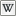# llLog

 LSL Portal
Function: float llLog( float val );
 265 Function ID 0 Forced Delay 10 Energy

Returns a float that is thenatural logarithm of val.
If val <= 0 return 0.0 instead.

 • float val

To get the base 10 logarithm use llLog10.

## Examples

```default
{
state_entry()
{
float num1 = llFrand(100.0);

llOwnerSay("The natural logarithm of " + (string)num1 + " is " + (string)llLog(num1));
}
}
```
```float findexp(float result, float base)
{
return llLog(result)/llLog(base);
}
default
{
touch_start(integer total_number)
{
llSay(0, (string)findexp(8.0,2.0));
//returns 3.0
}
}
```

```function float llLog( float val );